SSC BOARD PAPERS IMPORTANT TOPICS COVERED FOR BOARD EXAM 2024

### Practice Set 1.5 Linear Equations In Two Variables Class 10th Mathematics Part 1 MHB Solution

Practice Set 1.5
1. Two numbers differ by 3. The sum of twice the smaller number and thrice the greater…
2. Complete the following.
3. The sum of father’s age and twice the age of his son is 70. If we double the age of the…
4. The denominator of a fraction is 4 more than twice its numerator. Denominator becomes…
5. Two types of boxes A, B are to be placed in a truck having capacity of 10 tons. When…
6. Out of 1900 km, Vishal travelled some distance by bus and some by aeroplane. Bus…

###### Practice Set 1.5
Question 1.

Two numbers differ by 3. The sum of twice the smaller number and thrice the greater number is 19. Find the numbers.

Let the greater no. be x and smaller no. be x–3

As per given situation,

2(x–3) + 3(x) = 19

⇒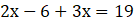⇒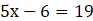⇒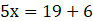⇒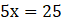⇒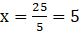∴ smaller no is x–3 ⇒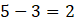Hence, The numbers are 5 and 2.

Question 2.

Complete the following.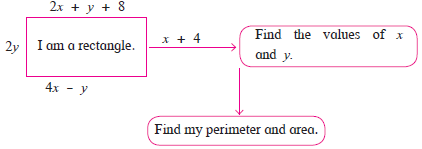Length of rectangle ⇒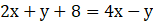⇒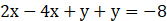⇒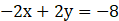⇒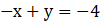……(I)

Breadth of the rectangle =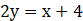⇒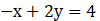……(II)

Equating Eq. I and II and change sign of Eq. II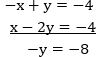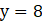Substituting y=8 in Eq.I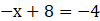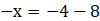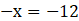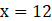Length =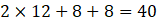Breadth =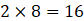Area = Length × breadth = 40× 16 = 640 sq. unit

Perimeter = 2(Length + Breadth) = 2(40+16) = 2(56) =112 unit.

Question 3.

The sum of father’s age and twice the age of his son is 70. If we double the age of the father and add it to the age of his son the sum is 95. Find their present ages.

Suppose father’s age(in years) be x and that son’s age be y.

Then,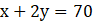…(I)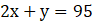…(II)

Multiply Eq.I by 2 and equate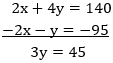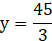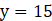Substituting y=15 in Eq.II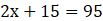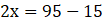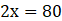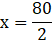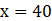∴ Son’s age is 15 years, father’s age is 40 years.

Question 4.

The denominator of a fraction is 4 more than twice its numerator. Denominator becomes 12 times the numerator, if both the numerator and the denominator are reduced by 6. Find the fraction.

Let the numerator and denominator of the fraction be x and y respectively.

Fraction =Given,

Denominator = 2(Numerator) + 4

⇒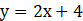⇒ 2x-y=(-4) …I

According to the given condition, we have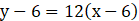⇒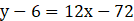⇒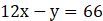…II

Equating Eq. I and II,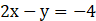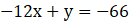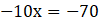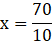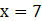Putting x = 7 in equation I, we get

⇒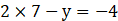⇒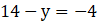⇒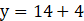⇒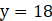Hence, required fraction =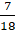Question 5.

Two types of boxes A, B are to be placed in a truck having capacity of 10 tons. When 150 boxes of type A and 100 boxes of type B are loaded in the truck, it weighes 10 tons. But when 260 boxes of type A are loaded in the truck, it can still accommodate 40 boxes of type B, so that it is fully loaded. Find the weight of each type of box.

A – 30kg, B – 55k

Let the weight of box ‘A’ = x kg

Let the Weight of box’B’ = y kg

According to question,

150 boxes of type A and 100 boxes of type B are loaded in the truck and it weighs 10tons.

∴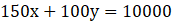[∵ 1ton = 1000kg]

⇒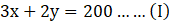260 boxes of type A are loaded in the truck, it can still accommodate 40 boxes of type B, still it weighs 10tons

∴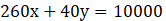[∵ 1ton = 1000kg]

⇒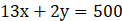……(II)

Solving Equation I and II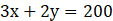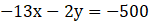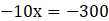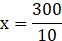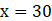Putting x=30 in Eq. I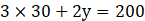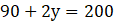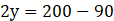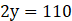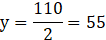Hence, A – 30kg, B – 55kg

Question 6.

Out of 1900 km, Vishal travelled some distance by bus and some by aeroplane. Bus travels with average speed 60 km/hr and the average speed of aeroplane is 700 km/hr. It takes 5 hours to complete the journey. Find the distance, Vishal travelled by bus.

Let the distance travelled by bus = x

Speed of bus = 60 km/hr
As,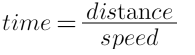Time taken travelling by bus =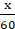Let the distance traveled by plane = y

As, total distance traveled was 1900 km
x + y = 1900

Distance traveled by plane = (1900–x)

Speed of plane = 700 km/hr

Time travelling by plane =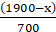Given,

Total time = 5 hours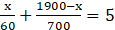⇒ 32x + 5700 = 10500
⇒ 32x = 4800
⇒ x = 150 km

and y = 1900 - x
= 1900 - 150 = 1750 km

Vishal travels 150km by bus and 1750 km by plane.